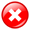# How To Read And Calculate Fractional Betting Odds On V9BET

Sports betting odds are quite region-dependent, so understanding fractional betting odds will give V9BET online bookie bettors an easier way to read and calculate profits when winning bets.

The following information from the V9betz experts of V9BET online bookie will help you better understand how fractional betting odds are calculated.

V9BET Online Bookie: Understanding Fractional Odds

You should recognize fractional odds right away if you see them represented as odds when a number is placed on top of another with a “/” in between them.

An illustration of a fractional odd is 4/5. The top number, or the one to the left, denotes your earnings, while the bottom number, or the one to the right, denotes the amount you bet.

In our example, the wager amount is 5 and the winning amount is 4, respectively. Simply said, if you stake \$5 and the chances are as stated, you will win \$4.

Others will frequently refer to the numbers as the numerator and the denominator, respectively. We frequently begin with the numerator and go on to the denominator when reading out loud fractional odds.

As a result, the chances of 4/5 and 3/1 are respectively read as 4 to 5 and 3 to 1 odds. 1/1 is frequently referred to as “evens” because both the stake and the winnings have equal worth.

The writing of fractional odds will always be in whole numbers. As a result, a bookmaker will write 7/2 instead of 3.5/1.

Since the United Kingdom and Ireland are the two countries where fractional odds are most frequently utilized, they are also known as UK odds.

Using V9BET Fractional Odds To Calculate Winnings

Although a lot of people think it’s difficult, figuring out your wins when employing fractional odds is rather simple. To demonstrate how simple it is to figure up your winnings, we’ll give you a few instances.

Consider that you want to wager on the MLS match using the V9BET bookie. The LA Galaxy is the team you are interested in, and it is now valued at 5/6 (-120).

You must apply the following straightforward formula:

(Numerator x Bet amount) ÷ Denominator = Winnings

So the following is how a \$100 wager on LA Galaxy might look:

(5 x \$100) ÷ 6 = \$83.33

Therefore, after the v9bet.com bookmaker has added your original \$100 stake, your winnings will be \$83.33 and your total payment will be \$183.33.

Due to the fact that all sportsbooks will compute your profits instantly, you don’t need to bother about learning any complicated formulae.

Once you’ve dealt with fractional odds for a while, you’ll eventually be able to determine your profits without using any mathematical methods.

Fractional Odds To Implied Probability Conversion

A percentage that displays the chance of an event occurring based on the odds provided by the sportsbook is known as implied probability. A higher percentage corresponding to a higher likelihood means that something is more likely to happen if it has a high probability.

A low probability, on the other hand, means that there are fewer chances of it happening, and as a result, the probability percentage will be smaller.

You should first take into account other factors before placing a wager, though, so that you don’t just do it because an event has a higher possibility.

Because there is just one conversion formula, converting fractional odds to implied probability is straightforward. Following is the formula to apply when determining the implied probability of an event:

Fractional Odds Implied Probability = (1 ÷ ((Fractional Odds) + 1)) x 100

Conclusion

The above sharing has helped bettors of V9BET online bookie at V9betz.com understand how Fractional betting odds are calculated, from which they can allocate bets and make more accurate betting decisions to generate more profits from betting.

``````